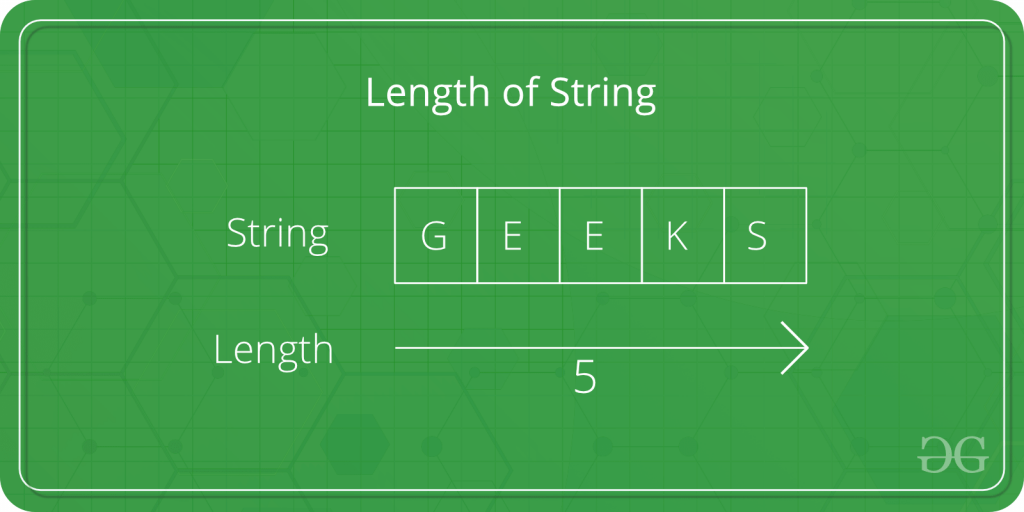Open in App
Not now

# C program to find the length of a string

• Difficulty Level : Basic
• Last Updated : 24 Jun, 2022

Given a string str. The task is to find the length of the string.Examples

```Input: str = "Geeks"
Output: Length of Str is : 5

Input: str = "GeeksforGeeks"
Output: Length of Str is : 13```

In the below program, to find the length of the string str, first the string is taken as input from the user using scanf in, and then the length of Str is calculated usingand usingmethod .
Below is the C program to find the length of the string.
Example 1: Using loop to calculate the length of string.

## C

 `// C program to find the length of string``#include ``#include ` `int` `main()``{``    ``char` `Str;``    ``int` `i;` `    ``printf``(``"Enter the String: "``);``    ``scanf``(``"%s"``, Str);` `    ``for` `(i = 0; Str[i] != ``'\0'``; ++i);` `    ``printf``(``"Length of Str is %d"``, i);` `    ``return` `0;``}`

Output:

```Enter the String: Geeks
Length of Str is 5```

Time Complexity: O(N)

Auxiliary Space: O(1)

Example 2: Using strlen() to find the length of the string.

## C

 `// C program to find the length of``// string using strlen function``#include ``#include ` `int` `main()``{``    ``char` `Str;``    ``int` `i;` `    ``printf``(``"Enter the String: "``);``    ``scanf``(``"%s"``, Str);` `    ``printf``(``"Length of Str is %ld"``, ``strlen``(Str));` `    ``return` `0;``}`

Output:

```Enter the String: Geeks
Length of Str is 5```

Time Complexity: O(1)

Auxiliary Space: O(1)

My Personal Notes arrow_drop_up